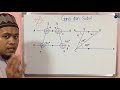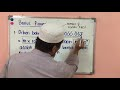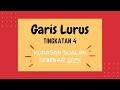#### Form 1##### MathematicsRational Numbers
Positive fractions and negative fractionsFactors and Multiples
Factors, prime factors and highest common factors (HCF)Ratio, Rates and Proportion
Ratios, rates and proportionsAlgebraic Expressions
Variables and algebraic expressionsVariables and algebraic expressionsLinear Inequalities
InequalitiesLines and Angles
Lines and anglesLines and angles#### Form 2##### MathematicsPatterns and Sequences
Patterns and SequencesPolygons
Regular PolygonsRegular PolygonsRegular PolygonsRegular PolygonsInterior Angles and Exterior Angles of PolygonsInterior Angles and Exterior Angles of PolygonsInterior Angles and Exterior Angles of PolygonsInterior Angles and Exterior Angles of PolygonsCircles
Circumference and Area of a CircleCircumference and Area of a CircleCoordinates
Midpoint in the Cartesian Coordinate System#### Form 3##### MathematicsIndices
Index NotationIndex NotationLaw of IndicesLaw of IndicesLaw of IndicesLaw of IndicesLaw of IndicesLaw of IndicesLaw of IndicesLaw of IndicesStandard Form
Standard FormStraight Lines
Straight Lines#### Form 4##### MathematicsNumber Bases
Number BaseNumber BaseProbability of Combined Events
Combined EventsApplication of the Probability of Combined Events#### Form 5##### MathematicsMatrices
Basic Operation on MatricesBasic Operation on MatricesBasic Operation on MatricesBasic Operation on Matrices